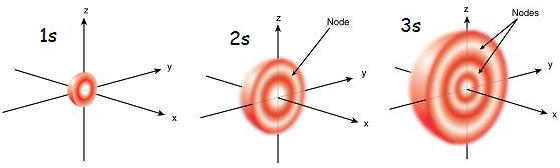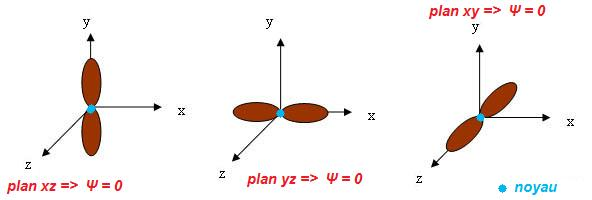# Difference Between S Orbital and P Orbital

## Main Difference – S Orbital vs P Orbital

An atom is composed of electrons that are in continuous movement in any direction around the nucleus. Since they are in movement around the nucleus, we cannot determine the exact position of that electron at a given moment. We can only guess the probability of an electron to be in a position. This phenomenon is called the Heisenberg Uncertainty Principle. According to these probabilities, the regions where an electron can be found at the highest probability are explained by the term orbital. There can be different orbitals according to the energies and movements of electrons around the nucleus. S orbital and p orbital are two such orbitals. The main difference between s orbital and p orbital is that s orbitals are spherical shaped whereas p orbitals are dumbbell shaped.

### Key Areas Covered

1. What is S Orbital
– Definition, Shape and Structural Properties
2. What is P Orbital
– Definition, Shape and Structural Properties
3. What are the Similarities Between S Orbital and P Orbital
– Outline of Common Features
4. What is the Difference Between S Orbital and P Orbital
– Comparison of Key Differences

Key Terms: Atom, Heisenberg Uncertainty Principle, Orbitals, P Orbital, Probability, S Orbital## What is S Orbital

S orbital is an atomic orbital that has a spherical shape. It has the lowest energy when compared to other atomic orbitals. Each electron shell has at least one s orbital. S orbital is the simplest atomic orbital among other orbitals. One s orbital can hold a maximum of two electrons. S orbitals have no sub-orbitals. The letter “s” stands for “sharp.” This orbital has been thus named considering the angular momentum of the electrons in that orbital. Since atomic orbitals are composed of a definite energy level (energy is quantized), they are given a quantum number. S orbital assigns the angular momentum quantum number of an atom.Figure 1: The size of the s orbital increases with the increasing of principle quantum number

The two electrons in the s orbital have opposite spins. S orbitals are involved in chemical bonding. They can take part in the formation of sigma bonds. But these s orbitals cannot form pi bonds. The spherical shape tells us the most probable region where the electrons can be found. S orbitals have no angular nodes. Therefore, the angular momentum quantum number of s orbital is 0.

S orbital has the lowest energy among all other orbitals in the same electron shell. At higher electron shells (principal quantum number = n), the s orbital has a lower energy than that of d orbitals in the nearest lower shell (n-1). The size of the s orbital sphere increase with the increasing of principle quantum number.

## What is P Orbital

P orbital is an atomic orbital having a dumbbell shape. P orbitals have a higher energy than that of s orbitals. The letter “p” stands for “principal.” It describes the angular momentum of electrons in the p orbital. One p orbital can hold a maximum of 6 electrons. These electrons occupy subatomic orbitals. One subatomic orbital can only hold a maximum of two electrons. Therefore, one p orbital has three subatomic orbitals. They are named as px, py and pz. In general, all these are called p orbitals.Figure 2: Shapes and Orientations of Three P Orbitals

The three sub orbitals of p orbital are different from each other according to the orientation of these orbitals in an atom. However, they are similar in their shape. All these suborbitals are dumbbell shaped. One special feature about p orbital is that it is composed of an angular node. Therefore, the angular momentum quantum number of p orbital is 1.

Except for the electron shell having principal quantum number 1, all other electron shells are composed of p orbitals. The size of the p orbitals increases with increasing principal quantum number. One p orbital has two lobes. These lobes are symmetrical along their axis. These p orbitals are involved in chemical bonding. They can form either sigma bonds or pi bonds. P sub orbitals in the horizontal orientation can for, sigma bonds. Other two suborbitals are involved pi bonding.

## Similarities Between S Orbital and P Orbital

• S orbital and P orbital are types of atomic orbitals.
• Both terms describe the angular momentum of electrons in that orbital.
• Both orbitals are involved in sigma bonding.

## Difference Between S Orbital and P Orbital

### Definition

S Orbital: S orbital is an atomic orbital that has a spherical shape.

P Orbital: P orbital is an atomic orbital that has a dumbbell shape.

### Energy Level

S Orbital: S orbitals have the lowest energy levels.

P Orbital: P orbitals have a higher energy than s orbitals.

### Angular Nodes

S Orbital: s orbitals have no angular nodes.

P Orbital: p orbitals have angular nodes

### Maximum Number of Electrons

S Orbital: The maximum number of electrons that an s orbital can hold is 2.

P Orbital: The maximum number of electrons that a p orbital can hold is 6.

### Sub orbitals

S Orbital: There are no sub orbitals in s orbitals.

P Orbital: There are 3 sub orbitals in p orbital.

### Angular Momentum Quantum Number

S Orbital: The angular momentum quantum number of s orbital is 0.

P Orbital: The angular momentum quantum number of p orbital is 1.

### Lobes

S Orbital: There are no lobes in s orbitals.

P Orbital: There are lobes present in p orbitals.

### Conclusion

Both s orbitals and p orbitals are atomic orbitals. These orbitals indicate the most probable region where we can find an electron of that atom. The main difference between s orbital and p orbital is that s orbitals are spherical shaped whereas p orbitals are dumbbell shaped.

##### References:

1. Libretexts. “Atomic Orbitals.” Chemistry LibreTexts, Libretexts, 3 Nov. 2015, Available here. Accessed 31 Aug. 2017.
2. Helmenstine, Ph.D. Anne Marie. “What Is a P Orbital?” ThoughtCo, Available here. Accessed 31 Aug. 2017.
3. Helmenstine, Ph.D. Anne Marie. “What s p d f Mean in Chemistry.” ThoughtCo, Available here. Accessed 31 Aug. 2017.

##### Image Courtesy:

1. “S orbitals” By CK-12 Foundation – File:High School Chemistry.pdf, page 265 (CC BY-SA 3.0) via Commons Wikimedia
2. “Orbitale-p” By Medenor – Own work (Public Domain) via Commons Wikimedia